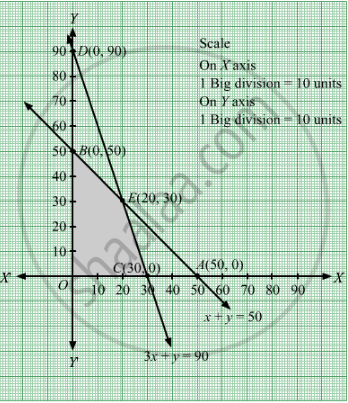# Solved the Following Linear Programming Problem Graphically: Maximize Z = 60x + 15y Subject to Constraints X + Y ≤ 50 3 X + Y ≤ 90 X , Y ≥ 0 - Mathematics

Solved the following linear programming problem graphically:
Maximize Z = 60x + 15y
Subject to constraints

$x + y \leq 50$
$3x + y \leq 90$
$x, y \geq 0$

#### Solution

We have to maximize Z = 60x + 15y
First, we will convert the given inequations into equations, we obtain the following equations:
x y = 50, 3x y = 90, x = 0 and y = 0

Region represented by x y  ≤ 50:
The line x y  = 50 meets the coordinate axes at A(50,0) and B(0,50) respectively. By joining these points we obtain the line 3x + 5y = 15.
Clearly (0,0) satisfies the inequation x y  ≤ 50. So,the region containing the origin represents the solution set of the inequation x y  ≤ 50.

Region represented by 3x y ≤ 90:
The line 3x y = 90 meets the coordinate axes at C(30, 0) and D(0, 90) respectively. By joining these points we obtain the line 3x y = 90.
Clearly (0,0) satisfies the inequation 3x y ≤ 90. So,the region containing the origin represents the solution set of the inequation 3x y ≤ 90.

Region represented by x ≥ 0 and y ≥ 0:
Since, every point in the first quadrant satisfies these inequations. So, the first quadrant is the region represented by the inequations x ≥ 0, and ≥ 0.

The feasible region determined by the system of constraints, x y  ≤ 50, 3x y ≤ 90, x ≥ 0, and y ≥ 0, are as follows.The corner points of the feasible region are O(0, 0), C(30, 0),

$E\left( 20, 30 \right)$ and B(0, 50).

The values of Z at these corner points are as follows.

 Corner point Z = 60x + 15y O(0, 0) 60 × 0 + 15 × 0 = 0 C(30, 0) 60 × 30 + 15 × 0 = 1800 $E\left( 20, 30 \right)$ 60 × 20 + 15 × 30 =1650 B(0, 50) 60 × 0 + 15 × 50 = 750

Therefore, the maximum value of Z is $1800 \text{ at the point } \left( 30, 0 \right)$ Hence, x = 30 and y = 0 is the optimal solution of the given LPP.
Thus, the optimal value of Z is 1800.

Concept: Graphical Method of Solving Linear Programming Problems
Is there an error in this question or solution?

#### APPEARS IN

RD Sharma Class 12 Maths
Chapter 30 Linear programming
Exercise 30.2 | Q 25 | Page 33